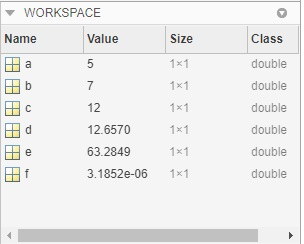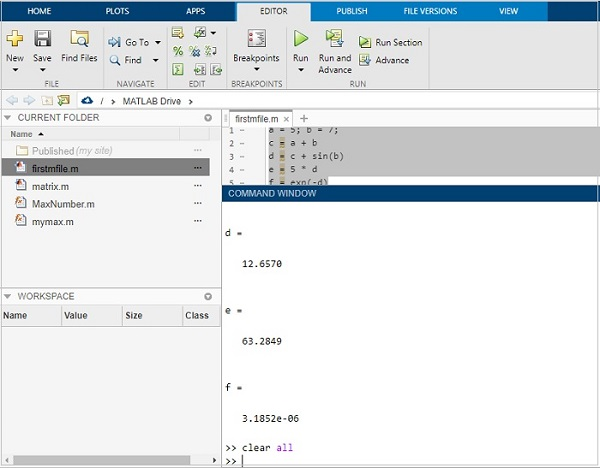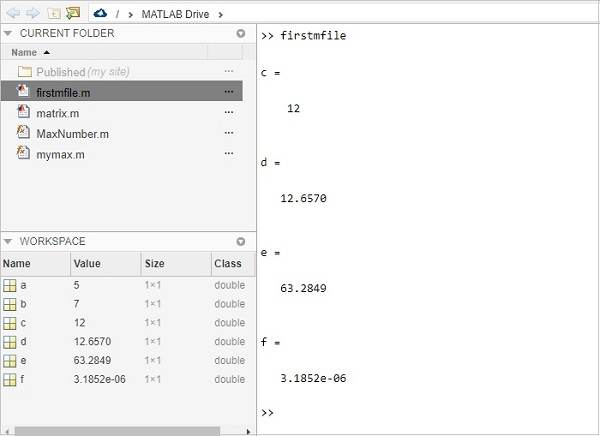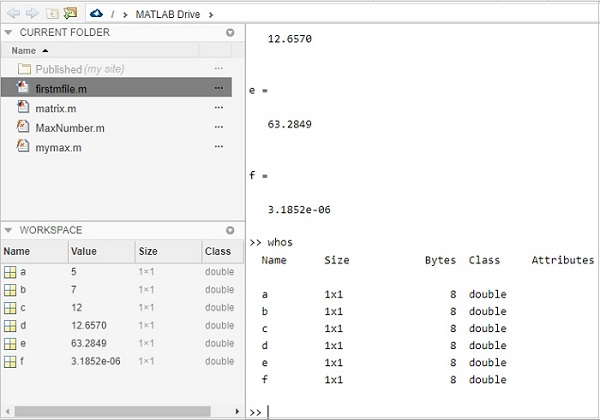# Matlab M-Files - Import Data

In this chapter, we will understand how to import data from an existing m-file in MATLAB.

Consider the m-file firstmfile.m. The contents of the file are as follows −

```a = 5; b = 7;
c = a + b
d = c + sin(b)
e = 5 * d
f = exp(-d)
```

When you execute the file, the data variables are available in the workspace, as shown below −Let us clear the workspace and the command prompt. Hence, we can now import the file and also check the data available.

To clear workspace, the command is clear all.The workspace is empty now.

Let us clean the command window by using clc command as shown below −We have an empty command window.

Now, type the m-file name as shown below. It will execute the code inside the fileThe workspace is loaded with all the variables that are used inside the file.

You can also check the details of the variables by using the whos command as shown below −